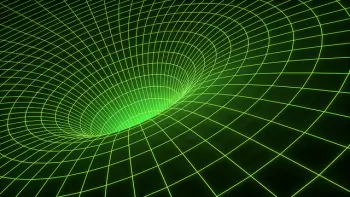# Energy Conservation LawIn physics, the law of conservation of energy is one of the most important conservation laws observed in nature. In its most studied and intuitive form, this law states that, although energy can be transformed and converted from one form to another, the total amount of it in an isolated system does not change over time.

However, in its most general sense, it does not seem correct to speak of the law, since in physics there are numerous laws related to the conservation of matter (mass) and energy: conservation of matter, mechanical energy, mass energy, the amount of movement, angular momentum, electric charge, etc. So in the scientific literature for the definition adopted is that of the principle of conservation of total energy (principle of conservation of energy), which include all possible forms of energy, including cataracts (after Einstein), but also the mass and amount of movement

The principle is also fulfilled in the field of quantum mechanics; in fact, Heisenberg's time-energy uncertainty principle does not have the same fundamental character as the counterpart that involves position and momentum, since a (universal) time operator is not defined in quantum mechanics.

However, the interpretation of thermodynamic phenomena in terms of statistical mechanics and the demonstration of the equivalence between heat and work and their constancy over time have extended the principle of conservation of energy outside the sphere to thermal phenomena. strictly mechanical. provided you consider all the ways in which energy can be produced.

## Particular Forms of the Law of Conservation of Energy

### Classical Mechanics

In Newtonian mechanics, a special case of the law of conservation of energy is formulated: the Law of conservation of mechanical energy, which sounds as follows: “The total mechanical energy of a closed system of bodies, among which only act the conservative forces, remain constant. ”

Simply put, in the absence of dissipative forces (for example, frictional forces), mechanical energy does not come out of nowhere and cannot disappear anywhere.

In a nuclear power plant, for example, the mechanical energy that drives the turbines is not generated from nothing, it comes from the thermal energy contained in the water vapor. Previously, this same energy was internal energy of atoms, specifically nuclear energy.

### Law of Conservation of Energy in Thermodynamics

In thermodynamics, historically, the law of conservation is formulated as the first principle of thermodynamics: “The change in the internal energy of a thermodynamic system during its transition from one state to another is equal to the sum of the work of the external forces in the system and the amount of heat transferred to the system, and it does not depend on the way in which this transition is performed. ” Or alternatively: "The amount of heat received by the system is used to change its internal energy and perform work against external forces."

The law of conservation of energy, in particular, establishes that there are no perpetual motion machines of the first type, that is, such processes are impossible, whose only result would be the production of work without any change in other bodies.

### Hydrodynamics

In the hydrodynamics of an ideal fluid, the law of conservation of energy is traditionally formulated in the form of the Bernoulli equation: the sum remains constant along the streamlines.

### Electrodynamics

In electrodynamics, the law of conservation of energy is historically formulated in the form of the Poynting theorem (sometimes also called the Umov - Poynting theorem), which relates the density of the flow of electromagnetic energy with the density of electromagnetic energy and the density of Joule's losses. Verbally, the theorem can be formulated as follows: “A change in electromagnetic energy enclosed in a certain volume over a certain time interval is equal to the flow of electromagnetic energy through the surface that limits a given volume and the amount of thermal energy released in a given volume taken with the opposite sign. ”

### Nonlinear Optics

In nonlinear optics, the propagation of optical (and usually electromagnetic) radiation in a medium is considered taking into account the multicantic interaction of this radiation with the substance of the medium. In particular, a wide range of studies is dedicated to the problems of the so-called three and four wave interactions in which three or four quanta of radiation interact, respectively. Since each individual act of such interaction obeys the laws of conservation of energy and momentum, it is possible to formulate fairly general relationships between the macroscopic parameters of the interacting waves. These relationships are called Manly-Rowe relationships.

### Relativistic Mechanics

In relativistic mechanics, the concept of an energy moment of 4 vectors (or only four moments) is introduced. Its introduction allows us to write the laws of conservation of the canonical moment and energy in a single form, which, in addition, is Lorentz-covariant, that is, it does not change during the transition from one inertial frame of reference to another.

### General Relativity

Being a generalization of the special theory of relativity, the general theory of relativity uses a generalization of the four-moment concept: the energy-momentum tensor. The conservation law is formulated for the energy-momentum tensor of the system.

In the general theory of relativity, the law of conservation of energy, strictly speaking, is fulfilled only locally. This is due to the fact that this law is a consequence of the uniformity of time, while in the general theory of relativity time is heterogeneous and undergoes changes depending on the presence of bodies and fields in space-time. It should be noted that with a correctly defined pseudotensor of the energy moment of the gravitational field, it is possible to achieve the conservation of the complete energy of the bodies and fields that interact gravitationally, including the gravitational. However, at this time there is no universally accepted way of introducing the energy of the gravitational field, since all the proposed options have one or another disadvantage. For example,